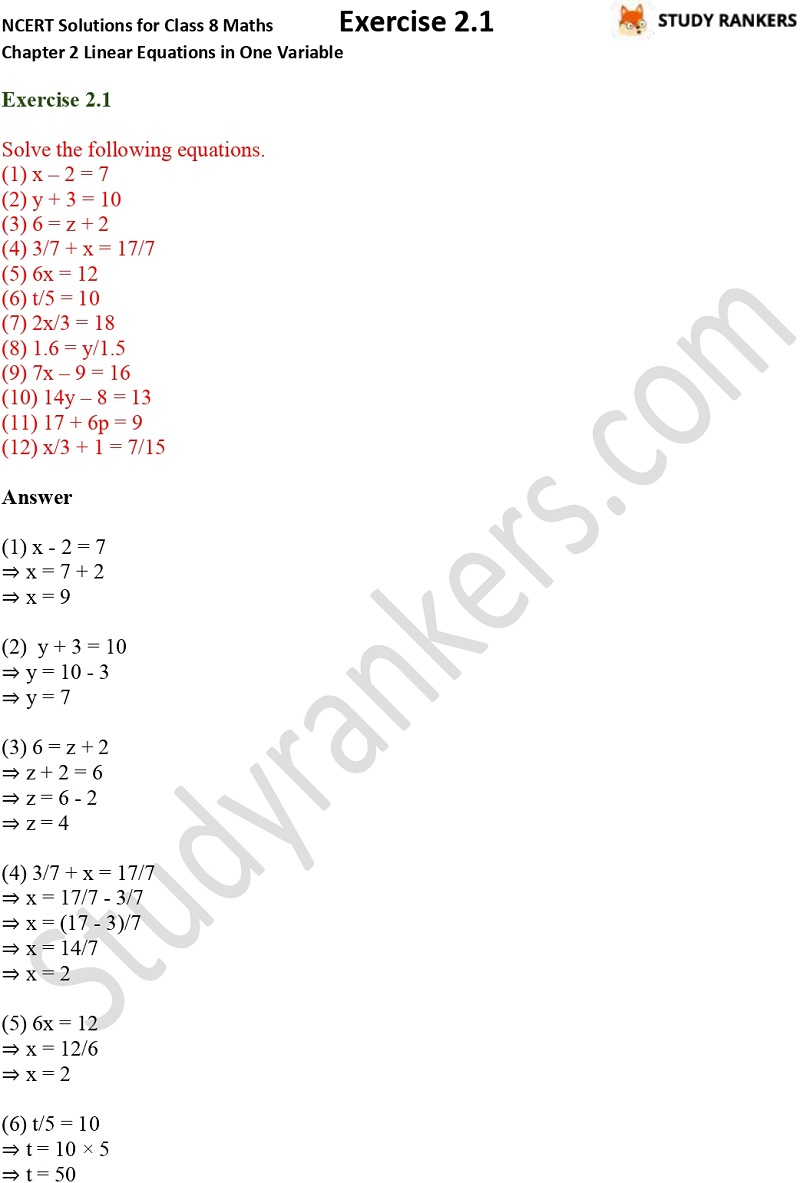>

## NCERT Solutions for Class 8 Maths Chapter 2 Linear Equations in One Variable Exercise 2.1

NCERT Solutions for Class 8 Maths Chapter 1 Rational Numbers Exercise 1.3 will help you in completing homework. Class 8 Maths NCERT Solutions is very helpful in strengthening you basic skills and knowing important points and concepts of the chapters. Studyrankers subject matter experts has prepared detailed and accurate NCERT questions and answers.

Exercise 2.1 has only one question which is about solving the equations.X